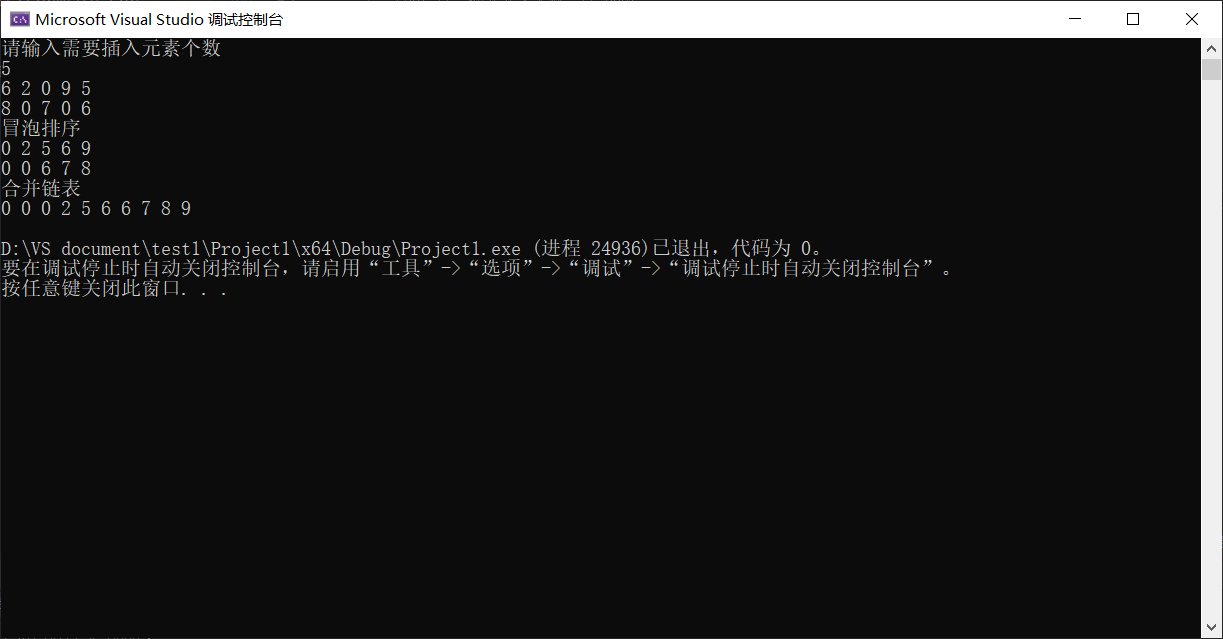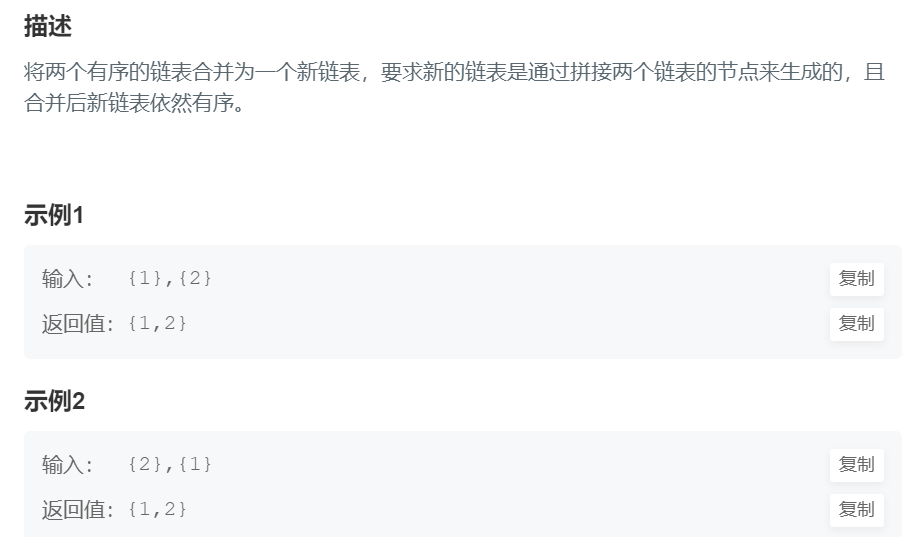• c++实现两有序链表合并成一个新链表
千次阅读
2016-11-22 16:28:04
struct Node
{
int x;
Node * next;
Node(int x1,Node * next1)
{
x = x1;
next = next1;
}
};

//--两个有序链表合并-假设排列方式为从小到大--
{
Node * MyPos = returnHead;// 标记新链表的最后一个位置
else
while(1)
{
{
break;
{
break;
}
{
}else
{
}
}

}




更多相关内容
• C++版本将两个有序链表合并一个新的有序链表并返回原理及代码实现
• 输入链表A 与B（空格分隔），说输入的数字序列可以无序 最后合并成一个有序的列表！MFC可视化编程
• 合并两个有序的顺序，使用语言是C++，数据结构里的一个基础的算法。

包括定义的链表，插入数据，及输出打印

#include <iostream>
#include <vector>
#include <numeric>
#include<algorithm>
using namespace std;
struct Node {
int x;
Node* next;
Node(int x1, Node* next1) {
x = x1;
next = next1;
}
};
//--两个有序链表合并-假设排列方式为从小到大--
}
else
while (1) {
{
break;
}
else if (head2 == nullptr) {
break;
}
}
else {
}
}
}
int main() {
Node* t;
int n, a;        //n为链表的长度
//a为每个结点的数据
cin >> n;
q = NULL;
for (int i = 0; i < n; i++) {
cin >> a;
p = (Node*)malloc(sizeof(Node));
p->x = a;
p->next = NULL;
}
else {
q->next = p;
}
q = p;
}
//--------------------------------//--------------------------------
//--------------------------------//--------------------------------
q = NULL;
for (int i = 0; i < n; i++) {
cin >> a;
p = (Node*)malloc(sizeof(Node));
p->x = a;
p->next = NULL;
}
else {
q->next = p;
}
q = p;
}
while (t != NULL) {
cout << t->x;          //打印合并后的链表
t = t->next;
}
return 0;
}

展开全文链表
• 虽然题目的意思是合并两个有序链表，但是要完全实现题目的意思首先我们需要先生成两个有序链表。种简单的方式是建立两个链表然后手动有序赋值。但是感觉这样不是很好，所以这里我选择随机生成然后自动排序的方式...

将两个升序链表合并为一个新的 升序 链表并返回。新链表是通过拼接给定的两个链表的所有节点组成的。

解这题之前看到一篇讲链表基本使用的文章，写的很详细。后面代码部分也是参考的这篇文章，可以看一下：

https://www.jb51.net/article/214771.htm


虽然题目的意思是合并两个有序链表，但是要完全实现题目的意思首先我们需要先生成两个有序链表。一种简单的方式是建立两个链表然后手动有序赋值。但是感觉这样不是很好，所以这里我选择随机生成然后自动排序的方式生成两个链表。：

## 1.随机生成一个链表

void InitList(Node*& head) {
}
//插入函数
bool Listinsert(Node*& head, int i) {
//插入到前面的方法
int value;
value = rand() % 10, 1;
int j = 0;
//如果插入的位置不合法，直接返回错误提示
if (i<1 || i>head->value + 1)return false;
//得到插入位置的前一个结点
while (j < i - 1) {
L = L->next;
++j;
}
//s是一个临时结点
Node* s = new Node();
s->value = value;    //先对临时结点赋值
s->next = L->next;   //让临时结点下一个位置指向当前需要插入前一个结点的下一个位置
L->next = s;          //让前一个结点下一个位置指向临时结点，完成
//线性表的长度加一
return true;
}


InitList是初始化一个链表。Listinsert是向该链表中插入一定的数据。数据长度由int i决定。

## 2.链表排序

链表的排序在上面的那个链接中大佬给出了三种方式，这里我使用了他其中最简单的我们最常用的排序方式：冒泡排序

void Listsort(Node*& head) {
int i = 0;
int j = 0;
//用于变量链表
//作为一个临时量
Node* p;
Node* p1;
//如果链表为空直接返回

for (i = 0; i < head->value - 1; i++) {
for (j = 0; j < head->value - i - 1; j++) {
//得到两个值
p = L;
p1 = L->next;
//如果前面的那个比后面的那个大，就交换它们之间的是数据域
if (p->value > p1->value) {
Elemtype temp = p->value;
p->value = p1->value;
p1->value = temp;
}
L = L->next;
}
}
}


## 3.链表合并

将两个升序链表合并为一个新的升序链表并返回。

这个方法有两种，今天自己尝试了写了一下花费了点时间但是最后还是实现了其中一种：

void combination(Node*& head, Node*& list, Node*& list3)
{
Node* L2 = list;
Node* L3 = list3;
L1 = L1->next;
L2 = L2->next;
//L3 = L3->next;
while (L1 != NULL || L2 != NULL)
{
L3->next = new Node();
L3 = L3->next;
if (L1 != NULL && L2 != NULL)
{
if (L1->value > L2->value)
{
L3->value = L2->value;
L2 = L2->next;
}
else
{
L3->value = L1->value;
L1 = L1->next;
}
}
else if (L1 == NULL && L2 != NULL)
{
L3->value = L2->value;
L2 = L2->next;
/* if (L2 != nullptr)
{
L3->next = new Node();
L3 = L3->next;
}*/
}
else
{
L3->value = L1->value;
L1 = L1->next;
/*if (L1 != nullptr)
{
L3->next = new Node();
L3 = L3->next;
}*/
}

//print(list3);
//L3 = L3->next;
}

}


这个方法比较简单也比较好理解，就是从两个链表中顺序取数，然后比较大小。小的数字存入第三个链表，大的数字保留。然后从小的数字那个链表中重新取下一个数字过来比较。循环直到结束。

这里唯一需要注意的是考虑到最后几位的比较。因为有可能一个链表数字取完了另一个链表中仍然存在一定的数据。所以这里要判断一下是否一个链表为空而另一个链表不为空的情况。

完整代码实现：

#include<iostream>
#include<ctime>
#include<cstdlib>
//#include<windows.h>
#include<algorithm>
using namespace std;
typedef int Elemtype;
//链式结构，我们打算在链表中添加一个
//保存长度的头结点，加入这个结点可以方便我们对结点做一些
//基本的操作，结点保存的是线性表的长度
struct Node
{
//结点的值,如果是头结点，保存是链表的长度
Elemtype value;
//下一个结点的地址
Node* next;
};
//创建一个空链表，每个头结点就代表一个链表
}
//插入函数
bool Listinsert(Node*& head, int i) {
//插入到前面的方法
int value;
value = rand() % 10, 1;
int j = 0;
//如果插入的位置不合法，直接返回错误提示
if (i<1 || i>head->value + 1)return false;
//得到插入位置的前一个结点
while (j < i - 1) {
L = L->next;
++j;
}
//s是一个临时结点
Node* s = new Node();
s->value = value;    //先对临时结点赋值
s->next = L->next;   //让临时结点下一个位置指向当前需要插入前一个结点的下一个位置
L->next = s;          //让前一个结点下一个位置指向临时结点，完成
//线性表的长度加一
return true;
}
//线性表的排序,采用冒泡排序,直接遍历链表
int i = 0;
int j = 0;
//用于变量链表
//作为一个临时量
Node* p;
Node* p1;
//如果链表为空直接返回

for (i = 0; i < head->value - 1; i++) {
for (j = 0; j < head->value - i - 1; j++) {
//得到两个值
p = L;
p1 = L->next;
//如果前面的那个比后面的那个大，就交换它们之间的是数据域
if (p->value > p1->value) {
Elemtype temp = p->value;
p->value = p1->value;
p1->value = temp;
}
L = L->next;
}
}
}

void combination(Node*& head, Node*& list, Node*& list3)
{
Node* L2 = list;
Node* L3 = list3;
L1 = L1->next;
L2 = L2->next;
//L3 = L3->next;
while (L1 != NULL || L2 != NULL)
{
L3->next = new Node();
L3 = L3->next;
if (L1 != NULL && L2 != NULL)
{
if (L1->value > L2->value)
{
L3->value = L2->value;
L2 = L2->next;
}
else
{
L3->value = L1->value;
L1 = L1->next;
}
}
else if (L1 == NULL && L2 != NULL)
{
L3->value = L2->value;
L2 = L2->next;
}
else
{
L3->value = L1->value;
L1 = L1->next;
}

}

}
//线性表的排序,采用冒泡排序,直接遍历链表
//线性表的排序，交换结点
//输出我们只需要传入头结点，然后循环判断当前结点下一个结点是否为空，
//这样就可以输出所有内容
while (L->next) {
L = L->next;
cout << L->value << " ";
}
cout << endl;
}
int main() {
//链表的头结点，不存放任何值，首先初始化头结点
Node* list;
Node* list3;
srand((int)time(NULL));     //每次执行种子不同，生成不同的随机数
//创建一个链表
InitList(list);
InitList(list3);
int i;
cout << "请输入需要插入元素个数" << endl;
int n;
cin >> n;//5
//cout << "请输入" << n << "个值" << endl;
for (i = 0; i < n; i++) {
Elemtype temp;
temp = rand() % 10, 1;
if (!Listinsert(head, i + 1)) {
cout << "插入元素失败" << endl;
}
if (!Listinsert(list, i + 1)) {
cout << "插入元素失败" << endl;
}
}

print(list);

cout << "冒泡排序" << endl;
Listsort(list);
//cout << list << endl;
print(list);
cout << "合并链表" << endl;
print(list3);
//system("pause");
return 0;
}## 递归

这里还有另外一种看起来比较简洁的方法：递归

Node* mergeTwoSortedLinkListWithRecursion(Node* head1, Node* head2) {
}
}
//递归可以理解为之后的情况都处理好了 只需要解决好当前这步就行了
}
else {
}
}


这个代码没具体实现。这个方法应该是可行的。

参考：
https://www.jb51.net/article/193132.htm

https://blog.csdn.net/qq_42673507/article/details/91360021

https://www.jb51.net/article/214771.htm

https://leetcode-cn.com/problems/merge-two-sorted-lists/solution/he-bing-liang-ge-you-xu-lian-biao-by-leetcode-solu/

展开全文c语言 开发语言
• 两个有序顺序表合并有序顺序 C++实现 顺序 C++ C 数据结构 顺序的排序
• 两个有序的链表合并成一个链表，合并后的链表仍然是有序的，依次输出合并后的链表的元素值，并求出第奇数位置元素之和数据结构 线性表
• 对于给定的两个链表本身是有序的，我们可以逐个向前对比两个链表L1和L2的节点大小，选择其中节点值小的链接到新的链表上，然后当前链表节点和选中的链表的下一个节点继续比较，直到两个链表中的任何一个遍历完毕，再...对于给定的两个链表本身是有序的，我们可以逐个向前对比两个链表L1和L2的节点大小，选择其中节点值小的链接到新的链表上，然后当前链表节点和选中的链表的下一个节点继续比较，直到两个链表中的任何一个遍历完毕，再将另一个没有遍历完的链表节点直接连接到新的链表上。（两个链表长度可能不一致，所以最后需要判断）。

代码如下：

class Solution {
public:
/**
*
* @param l1 ListNode类
* @param l2 ListNode类
* @return ListNode类
*/
ListNode* mergeTwoLists(ListNode* l1, ListNode* l2) {
// write code here
if(l1==nullptr&&l2==nullptr) return nullptr;
while(l1!=nullptr&&l2!=nullptr)
{
if(l1->val<l2->val)
{
l1=l1->next;
}
else
{
l2=l2->next;
}
}
if(l1!=nullptr)
if(l2!=nullptr)
return res->next;
}
};

展开全文• 两个有序链表合并一个新的有序链表并返回。新链表是通过拼接给定的两个链表的所有节点组成的。 示例： 输入：1->2->4, 1->3->4 输出：1->1->2->3->4->4 /** * Definition for ...
• 两个升序链表合并一个新的 升序 链表并返回。新链表是通过拼接给定的两个链表的所有节点组成的。 输入：l1 = [1,2,4], l2 = [1,3,4] 输出：[1,1,2,3,4,4] 解法一：迭代 合并前： 合并后： class Solution { ...leetcode 算法 链表 单链表
• //将两个有序顺序表合并一个新的有序表，并由函数返回结果顺序表 typedef struct{ int data[MaxSize]; int Length; }SqList; //1.保证顺序表A+顺序表B的长度不超过MaxSize //2.两个指针分别指向A,B，判断指针...
• 有序的链表p1 和 p2 的头部比较值的大小，然后将比较后得出的头结点 插入到新链表的尾部，不断循环遍历，最后输出一个新链表 #include<stdio.h> #include<stdlib.h> #include<string.h> ...链表 c语言 数据结构
• 对于k个链表的合并，我们可以基于两个有序链表的合并思想，先将两个链表合并一个链表，再将得到的结果链表与第三个链表合并一个链表，以此类推，最终将k个链表合并一个有序链表。 本篇不在累述这种方法，对于...数据结构 链表
• 两个递增的有序链表合并一个递增的有序链表。要求结果链表仍使用原来两个链表的存储空间, 不另外占用其它的存储空间。中不允许有重复的数据。 [题目分析] 合并后的新用头指针Lc指向，pa和pb分别是链表La和Lb...链表 数据结构 算法
• 方法一：若要求不能对原始链表更改，则必须使用额外空间 ... /*先创建一个头结点 这里用任意的整数都可以 不一定用0 之后返回newHead->next 即可 该方法在很多时候都可以起到简化代码的作用 值得借鉴*/...
•数据结构 链表链表
• 有关于基于C++的数据结构两个顺序表合并有序表类的实现。
• 两个有序的链表合并一个有序链表，链表的大小是可变的
• //归并有序表La和Lb，生成新的有序表Lc //并且在归并之后删除La和Lb pa = La->next; pb = Lb->next; pre = La;//将pre作为链表合并后的新的头节点 while (pa&&pb)//la和lb任意条链表归并完毕...链表
• 分享一个大牛的人工智能教程。零基础！通俗易懂！风趣幽默！希望你也加入到人工智能的队伍中来！请点击http://www.captainbed.net /* * Created by Chimomo */ #include &lt;iostream&gt; #define ...数据结构
• 1.递归 2.迭代 #include<iostream> using namespace std; //结构。 struct ListNode { int val; ListNode* next; ListNode(int x) :val(x), next(NULL) {} }; class solution { public: ...链表 算法 数据结构
• 两个有序链表合并一个新的有序链表并返回。新链表是通过拼接给定的两个链表的所有节点组成的。 示例： 输入：1->2->4, 1->3->4 输出：1->1->2->3->4->4 思路： 从链表1中取出第......

# 两个表合并成一个有序表c++c++ 订阅